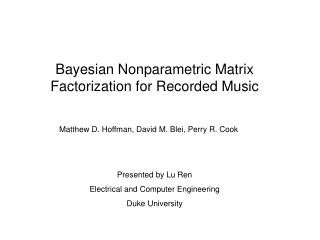Download PresentationBayesian Nonparametric Matrix Factorization for Recorded Music# Bayesian Nonparametric Matrix Factorization for Recorded Music - PowerPoint PPT Presentation

Download Presentation##### Bayesian Nonparametric Matrix Factorization for Recorded Music

Download Policy: Content on the Website is provided to you AS IS for your information and personal use and may not be sold / licensed / shared on other websites without getting consent from its author. While downloading, if for some reason you are not able to download a presentation, the publisher may have deleted the file from their server.

- - - - - - - - - - - - - - - - - - - - - - - - - - - E N D - - - - - - - - - - - - - - - - - - - - - - - - - - -
##### Presentation Transcript

1. Bayesian Nonparametric Matrix Factorization for Recorded Music Matthew D. Hoffman, David M. Blei, Perry R. Cook Presented by Lu Ren Electrical and Computer Engineering Duke University

2. Outline • Introduction • GaP-NMF Model • Variational Inference • Evaluation • Related Work • Conclusions

3. Introduction • Breaking audio spectrograms into separate sources of sound Identifying individual instruments and notes Predicting hidden or distorted signals Source separation previous work • Specifying the number of sources---Bayesian Nonparametric • Gamma Process Nonnegative Matrix Factorization (GaP-NMF) • Computational challenge: non-conjugate pairs of distributions • favor for spectrogram data, not for computational convenience • bigger variational family analytic coordinate ascent algorithm

4. GaP-NMF Model • Observation: Fourier power sepctrogram of an audio signal : M by N matrix of nonnegative reals : power at time window n and frequency bin m A window of 2(M-1) samples Squared magnitude in each frequency bin DFT Keep only the first M bins • Assume K static sound sources : describe these sources is the average amount of energy source k exhibits at frequency m : amplitude of each source changing over time is the gain of source k at time n

5. GaP-NMF Model Mixing K sound sources in the time domain (under certain assumptions), spectrogram is distributed1 Infer both the characters and number of latent audio sources : trunction level 1Abdallah & Plumbley (2004) and Fevotte et al. (2009)

6. GaP-NMF Model • As goes infinity, approximates an infinite sequence drawn from a gamma process • Number of elements greater than some is finite almost surely: • If is sufficiently large relative to , only a few elements of are substantially greater than 0. • Setting :

7. Variational Inference Variational distribution: expanded family Generalized Inverse-Gaussian (GIG): denotes a modified Bessel function of the second kind Gamma family is a special case of the GIG family where ,

8. Variational Inference Lower bound of GaP-NMF model: If : GIG family sufficient statistics: Gamma family sufficient statistics:

9. Variational Inference The likelihood term expands to: With Jensen’s inequality:

10. Variational Inference With a first order Taylor approximation: : an arbitrary positive point

11. Variational Inference • Tightening the likelihood bound • Optimizing the variational distributions For example:

12. Evaluation Compare GaP-NMF to two variations: 1. Finite Bayesian model 2. Finite non-Bayesian model Itakura-Saito Nonnegative Matrix Factorization (IS-NMF) : maximize the likelihood in the above fomula Compare with another two NMF algorithms: EU-NMF: minimize the sum of the squared Euclidean distance KL-NMF: minimize the generalized KL-divergence

13. Evaluation 1. Synthetic Data

14. Evaluation 2. Marginal Likelihood & Bandwidth Expansion

15. Evaluation 3. Blind Monophonic Source Separation

16. Conclusions • Related work • Bayesian nonparametric model GaP-NMF • Applicable to other types of audio